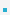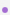7. Relational and Logical Operators

7.1 Relational OperatorsRelational operators compare two operands to produce a ’boolean’ result. In C any non-zero value (1    by convention) is considered to be ’true’ and 0 is considered to be false.Note that the "==" operator on float variables is tricky because of finite precisional.
 operator meaning examples > greater than 3 > 2; /* evaluates to 1 */ 2.99 > 3; /* evaluates to 0 */ >= greater than or equal to 3 >= 3; /* evaluates to 1 */ 2.99 >= 3; /* evaluates to 0 */ < less than 3<3; /*evaluates to 0 */ ’A’<’B’/*evaluates to 1*/ < less than or equal to 3<=3; /*evaluates to 1 */ 3.99<3 /*evaluates to 0 */ == equal to 3==3; /*evaluates to 1 */ ’A’==’a’/*evaluates to 0 */ != not equal to 3!=3; /*evaluates to 0 */ 2.99!=3 /*evaluates to 1 */

7.2 Logical OperatorsThe evaluation of an expression is discontinued if the value of a conditional expression can be    determined early. Be careful of any side effects in the code.Examples:
– (3==3) || ((c=getchar())==’y’). The second expression is not evaluated.
– (0) && ((x=x+1)>0) . The second expression is not evaluated.

 operator meaning examples && AND ((9/3)==3) && (2*3==6); /*evaluates to 1 */ (’A’==’a’) && (3==3) /*evaluates to 0 */ || OR 2==3 || ’A’==’A’; /*evaluates to 1 */ 2.99>=3 || 0 /*evaluates to 0 */ ! NOT !(3==3); /*evaluates to 0 */ !(2.99>=3) /*evaluates to 1 */

7.3 Increment and Decrement OperatorsIncrement and decrement are common arithmetic operation. C provides two short cuts for the same.Postfix
– x++ is a short cut for x=x+1
– x−− is a short cut for x=x−1
– y=x++ is a short cut for y=x; x=x+1. x is evaluated before it is incremented.
– y=x−− is a short cut for y=x; x=x−1. x is evaluated before it is decremented.Prefix
– ++x is a short cut for x=x+1
– −−x is a short cut for x=x−1
– y=++x is a short cut for x=x+1; y=x;. x is evaluate after it is incremented.
– y=−−x is a short cut for x=x−1; y=x;. x is evaluate after it is decremented.

7.4 Bitwise OperatorsAND is true only if both operands are true.OR is true if any operand is true.XOR is true if only one of the operand is true.

 operator meaning examples & AND 0x77 & 0x3; /*evaluates to 0x3 */ 0x77 & 0x0; /*evaluates to 0 */ | OR 0x700 | 0x33; /*evaluates to 0x733 */ 0x070 | 0 /*evaluates to 0x070 */ ^ XOR 0x770 ^ 0x773; /*evaluates to 0x3 */ 0x33 ^ 0x33; /*evaluates to 0 */ << left shift 0x01<<4; /*evaluates to 0x10 */ 1<<2; /*evaluates to 4 */ >> right shift 0x010>>4; /*evaluates to 0x01 */ 4>>1 /*evaluates to 2 */

7.5 Assignment OperatorsAnother common expression type found while programming in C is of the type var = var (op) expr
– x=x+1
– x=x*10
– x=x/2C provides compact assignment operators that can be used instead.
– x+=1 /*is the same as x=x+1*/
– x−=1 /*is the same as x=x−1*/
– x*=10 /*is the same as x=x*10 */
– x/=2 /* is the same as x=x/2
– x%=2 /*is the same as x=x%2

7.6 Conditional ExpressionA common pattern in C (and in most programming) languages is the following:
 if ( cond )         x = ; else         x = ;C provides syntactic sugar to express the same using the ternary operator ’?:’
 sign=x>0?1:−1; if    (x>0)      sign=1; else      sign=-1; isodd=x%2==1?1:0; if  (x%2==1)     isodd=1; else     isodd=0;Notice how the ternary operator makes the code shorter and easier to understand (syntactic sugar).

 이전페이지 / 8 / 다음페이지